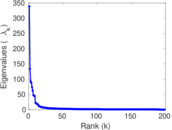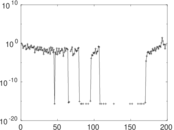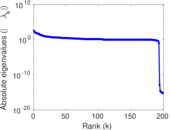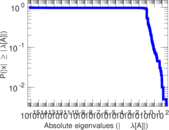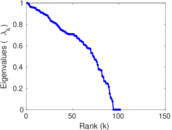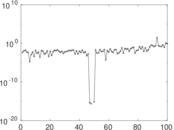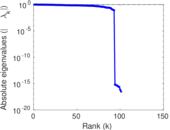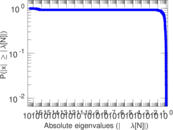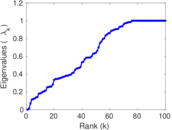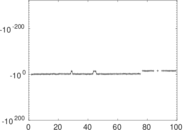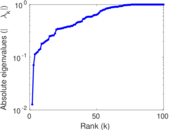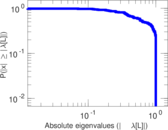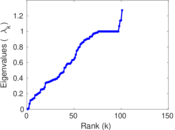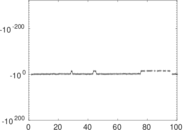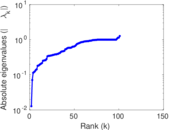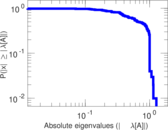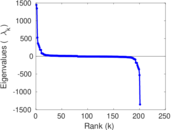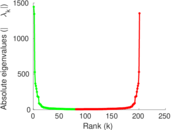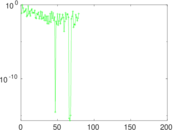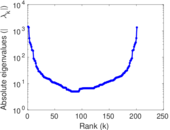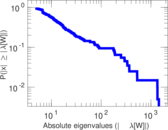# Wikibooks edits (et)

This is the bipartite edit network of the Estonian Wikibooks. It contains users and pages from the Estonian Wikibooks, connected by edit events. Each edge represents an edit. The dataset includes the timestamp of each edit.

 Code `bet` Internal name `edit-etwikibooks` Name Wikibooks edits (et) Data source http://dumps.wikimedia.org/ AvailabilityDataset is available for download Consistency checkDataset passed all tests Category Authorship network Dataset timestamp 2017-10-20 Node meaning User, article Edge meaning Edit Network formatBipartite, undirected Edge typeUnweighted, multiple edges Temporal dataEdges are annotated with timestamps

## Statistics

 Size n = 1,447 Left size n1 = 201 Right size n2 = 1,246 Volume m = 4,863 Unique edge count m̿ = 1,881 Wedge count s = 148,160 Claw count z = 13,646,886 Cross count x = 1,088,212,032 Square count q = 15,443 4-Tour count T4 = 721,098 Maximum degree dmax = 887 Maximum left degree d1max = 887 Maximum right degree d2max = 235 Average degree d = 6.721 49 Average left degree d1 = 24.194 0 Average right degree d2 = 3.902 89 Fill p = 0.007 510 60 Average edge multiplicity m̃ = 2.585 33 Size of LCC N = 1,177 Diameter δ = 14 50-Percentile effective diameter δ0.5 = 3.638 96 90-Percentile effective diameter δ0.9 = 6.859 06 Median distance δM = 4 Mean distance δm = 4.474 61 Gini coefficient G = 0.785 647 Balanced inequality ratio P = 0.177 874 Left balanced inequality ratio P1 = 0.103 023 Right balanced inequality ratio P2 = 0.249 229 Relative edge distribution entropy Her = 0.806 662 Power law exponent γ = 3.836 71 Tail power law exponent γt = 2.341 00 Tail power law exponent with p γ3 = 2.341 00 p-value p = 0.000 00 Left tail power law exponent with p γ3,1 = 1.811 00 Left p-value p1 = 0.085 000 0 Right tail power law exponent with p γ3,2 = 5.361 00 Right p-value p2 = 0.755 000 Degree assortativity ρ = −0.069 082 3 Degree assortativity p-value pρ = 0.002 720 05 Spectral norm α = 338.982 Algebraic connectivity a = 0.012 710 4 Spectral separation |λ1[A] / λ2[A]| = 2.533 16 Controllability C = 1,058 Relative controllability Cr = 0.732 687

## Plots

### Fruchterman–Reingold graph drawing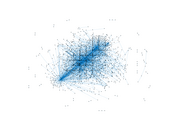### Degree distribution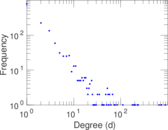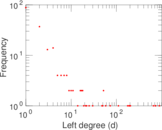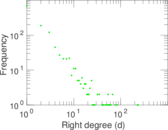### Cumulative degree distribution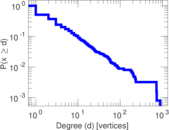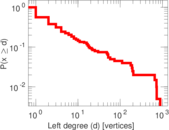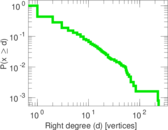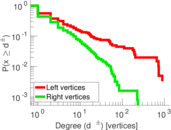### Lorenz curve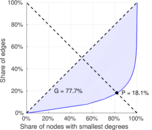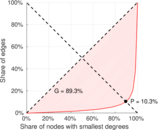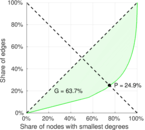### Spectral distribution of the adjacency matrix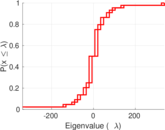### Spectral distribution of the normalized adjacency matrix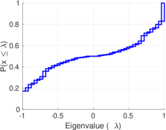### Spectral distribution of the Laplacian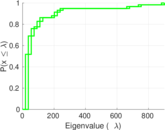### Spectral graph drawing based on the adjacency matrix### Spectral graph drawing based on the Laplacian### Spectral graph drawing based on the normalized adjacency matrix### Degree assortativity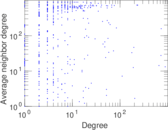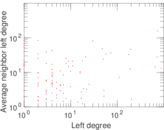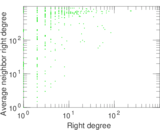### Zipf plot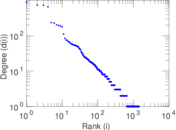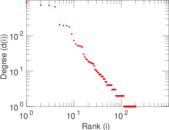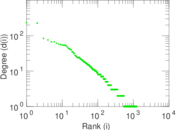### Hop distribution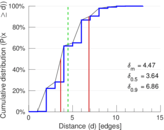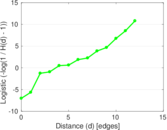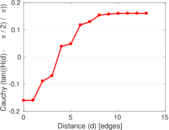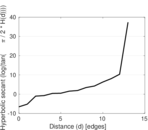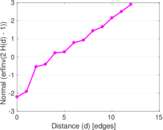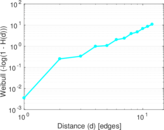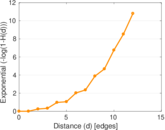### Double Laplacian graph drawing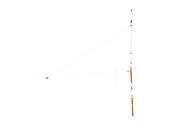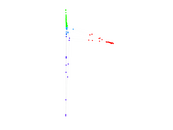### Delaunay graph drawing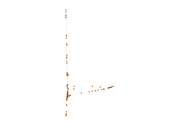### Edge weight/multiplicity distribution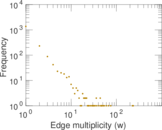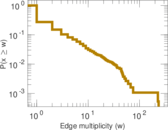### Temporal distribution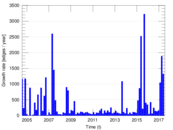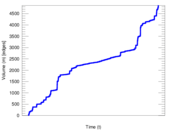### Temporal hop distribution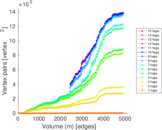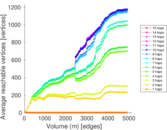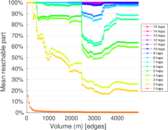### Diameter/density evolution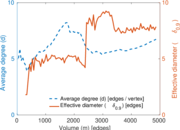### Matrix decompositions plots